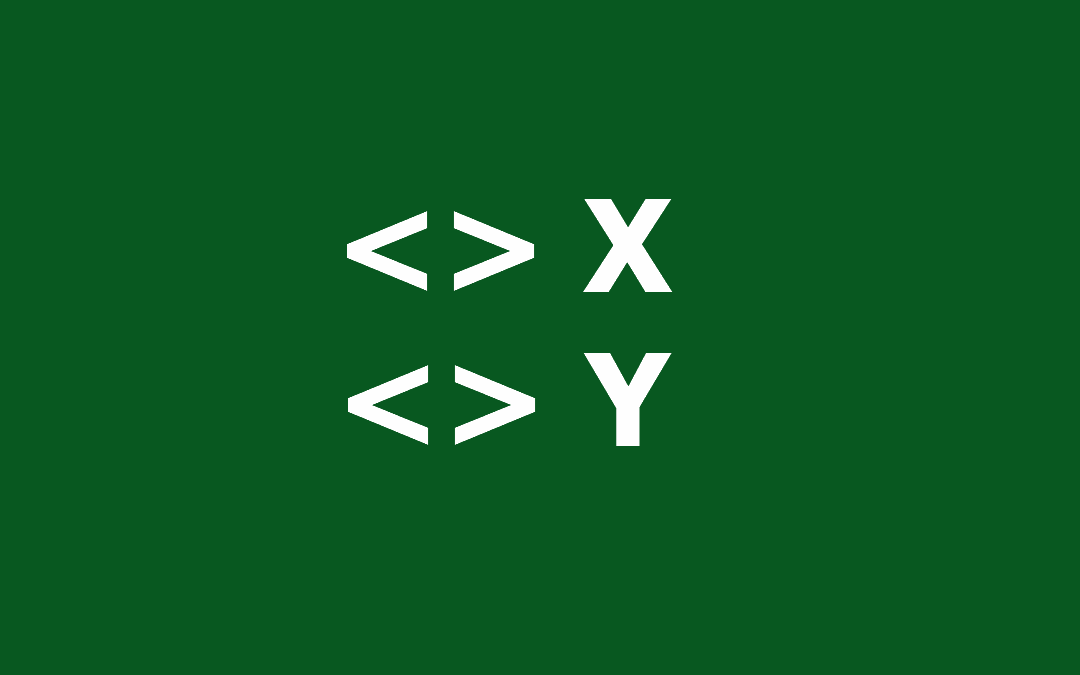## Function: VAR.S

The VAR.S function is a statistical function calculates and returns the variance of a sample. It is also a replacement for the outdated VAR function. The variance provides a general idea of the spread of data, and can be used to calculate the standard deviation or...## How to count the number of cells greater than the average in Excel

In this article, we are going to show you how to count the number of cells greater than the average in Excel. Download Workbook Formula =COUNTIFS(<numbers range>,”>“&AVERAGE(<numbers range>)) <numbers range>: The reference from the range of...## How to count the number of cells less than the average in Excel

In this article, we are going to show you how to count the number of cells that is less than the average of that data set in Excel. Download Workbook Formula =COUNTIFS(<numbers range>,”<“&AVERAGE(<numbers range>)) <numbers range>: The...## How to do a cell count if not equal to a variable or even an error code

You can use the COUNTIFS function to count the values in ranges that meet certain criteria. The criteria can contain a not equal logic as well. However, the COUNTIFS function requires individual arguments for each range-criteria pair. This requirement can make it...## How to count if not equal to x or y in Excel

The COUNTIFS function can count the values in multiple ranges with multiple criteria. The function counts each given range-criteria pair and combines the result with AND logical operator. Unfortunately, there is no quick and easy way to use an OR logic with the...## How to make Excel calculate faster

Excel is a fairly an efficient software given that it can perform complex calculations. However, depending on your system, it can also get pretty slow when running complicated calculations. How formulas are used, and size of the workbook are typically the culprit if...## Control Shift Enter Excel Shortcut (CSE) and Array Formulas

Control Shift Enter Excel shortcut, as known as CSE, is a key combination you can use to apply array functions.CSE abbreviation is also used for array formulas that are entered using the Control + Shift + Enter key combination when entering a formula. Array formulas...